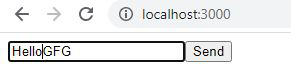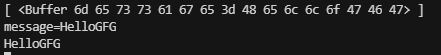Related Articles

# What is chunk in Node.js ?

• Difficulty Level : Basic
• Last Updated : 26 May, 2021

The data is a transfer from server to client for a particular request in the form of a stream. The stream contains chunks. A chunk is a fragment of the data that is sent by the client to server all chunks concepts to each other to make a buffer of the stream then the buffer is converted into meaning full data. In this article, we will discuss how to send chunks from the request body to the server. The request object is used for handling the chunks of data.

Syntax:

`request.on('eventName',callback)`

Parameters: This function accepts the following two parameters:

• eventName: It is the name of the event that fired
• callback: It is the Callback function i.e Event handler of the particular event.

Return type: The return type of this method is void.

Example: Create an index.js file with the following code.

## index.js

 `// Importing http libraries``const http = require(``'http'``);`` ` `// Creating a server``const server = http.createServer((req, res) => {``    ``const url = req.url;``    ``const method = req.method;`` ` `    ``if` `(url === ``'/'``) {``        ``// Sending the response``        ``res.write(``''``);``        ``res.write(``'Enter Message'``);``        ``res.write(`
``    ````    ```);``        ``res.write(``''``);``        ``return` `res.end();``    ``}`` ` `    ``// Handling different routes for different type request``    ``if` `(url === ``'/message'` `&& method === ``'POST'``) {``        ``const body = [];`` ` `        ``req.on(``'data'``, (chunk) => {`` ` `            ``// Storing the chunk data``            ``body.push(chunk);``            ``console.log(body)``        ``});`` ` `        ``req.on(``'end'``, () => {`` ` `            ``// Parsing the chunk data``            ``const parsedBody = Buffer.concat(body).toString();``            ``const message = parsedBody.split(``'='``);``             ` `            ``// Printing the data``            ``console.log(message);``        ``});`` ` `        ``res.statusCode = 302;``        ``res.setHeader(``'Location'``, ``'/'``);``        ``return` `res.end();``    ``}``});`` ` `// Starting the server``server.listen(3000);`

Run index.js file using below command:

`node index.js`

Now open a browser and go to http://localhost:3000, you will see the following output.Console Output:My Personal Notes arrow_drop_up